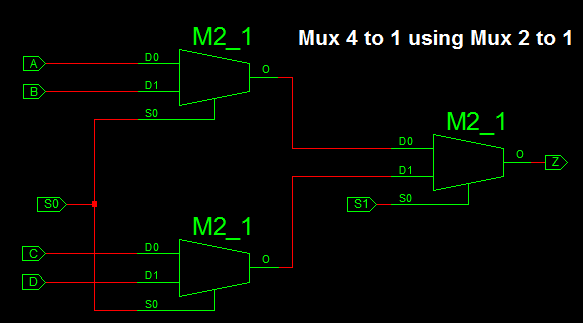# VHDL Component and Port Map Tutorial

### VHDL Port Map and Component

Component is a reusable VHDL module which can be declared with in another digital logic circuit using Component declaration of the VHDL Code. This helps to implement hierarchical design at ease.

Instead of coding a complex design in single VHDL Code. we can divide the code in to sub modules as component and combine them using Port Map technique.

VHDL Port Map is the Process of mapping the input/ Output Ports of Component in Main Module.

### Port Map Block DiagramThere are 2 ways we can Port Map the Component in VHDL Code. They are

• Positional Port Map
• Nominal Port Map

Positional Port Map maps the formal in/out port location with actual in/out port without changing its location.

For example:

```
Component and_gate

port( x,y: in std_logic;

z: out std_logic);

end component;

a1: and_gate port map(a,b,out);

```

Nominal Port Map assign the formal parameter with actual parameter as shown below.

`a1: and_gate port map(a => x, b => y, out =>z);`

Let’s Create sample Component and Port Map in Main VHDL Module.

Here we Construct 2 to 1 Mux and Port Map the 2 to 1 mux component to implement 4 to 1 mux.### Port Map Example: VHDL Code for 2 to 1 Mux

```
library IEEE;
use IEEE.STD_LOGIC_1164.ALL;

entity sub_module is
port(x,y : in STD_LOGIC;
s: in STD_LOGIC;
z: out STD_LOGIC);
end sub_module ;

architecture Behavioral of sub_module is

begin

process (x,y,s) is
begin
if (s ='0') then
z <= x;
else
z <= y;
end if;
end process;

end Behavioral;

```

The 2 to 1 mux can be port mapped in the 4 to 1 mux VHDL code by declaring it as component.

### VHDL Code for 4 to 1 mux using 2 to 1 mux

```
library IEEE;
use IEEE.STD_LOGIC_1164.ALL;

entity top_module is
port(

A,B,C,D : in STD_LOGIC;
S0,S1: in STD_LOGIC;
Z: out STD_LOGIC
);
end top_module ;

architecture Behavioral of top_module is
component sub_module
port(x,y : in STD_LOGIC;
s: in STD_LOGIC;
z: out STD_LOGIC);
end component;
signal m1, m2: std_logic;

begin
c1: sub_module port map(A,B,S0,m1);
c2: sub_module port map(C,D,S0,m2);
c3: sub_module port map(m1,m2,S1,Z);

end Behavioral;

```

In the above code we implemented positional port mapping technique by mapping at exact port location.

same can be declared with nominal Port mapping as shown below

```
c1: sub_module port map(A => x, B => y , S0  => s,  m1 => z);
c2: sub_module port map(C => x, D => y, S0 => s, m2 => z);
c3: sub_module port map(m1 => x, m2 => y, S1 => s, Z => z);

```

### 6 thoughts on “VHDL Component and Port Map Tutorial”

1.its so good best wishes. M.Mahdi

2.This should be other way i think especially portmap

c1: sub_module port map(A => x, B => y , S0 => s, m1 => z);
(x => A, y => B , s => S0, z => m1)

c2: sub_module port map(C => x, D => y, S0 => s, m2 => z);

c3: sub_module port map(m1 => x, m2 => y, S1 => s, Z => z);

3.This is the most helpful example of how to use components and port maps that I have seen. Thank you.

4.5.6.